Next: Transformers Up: Inductance Previous: The Circuit

## The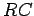Circuit

Let us now discuss a topic which, admittedly, has nothing whatsoever to do with inductors, but is mathematically so similar to the topic just discussed that it seems sensible to consider it at this point.

Consider a circuit in which a battery of emfis connected in series with a capacitor of capacitance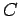, and a resistor of resistance. For fairly obvious reasons, such a circuit is generally referred to as ancircuit. In steady-state, the charge on the positive plate of the capacitor is given by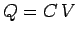, and zero current flows around the circuit (since current cannot flow across the insulating gap between the capacitor plates).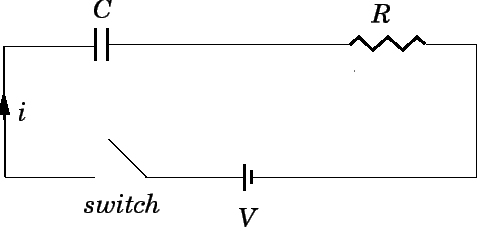Let us now introduce a switch into the circuit, as shown in Fig. 49. Suppose that the switch is initially open, but is suddenly closed at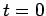. It is assumed that the capacitor plates are uncharged when the switch is thrown. We expect a transient current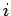to flow around the circuit until the chargeon the positive plate of the capacitor attains its final steady-state value. But, how long does this process take?

The potential differencebetween the positive and negative plates of the capacitor is given by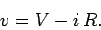(268)

In other words, the potential difference between the plates is the emf of the battery minus the potential drop across the resistor. The chargeon the positive plate of the capacitor is written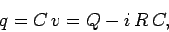(269)

whereis the final charge. Now, ifis the instantaneous current flowing around the circuit, then in a short time interval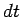the charge on the positive plate of the capacitor increases by a small amount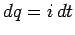(since all of the charge which flows around the circuit must accumulate on the plates of the capacitor). It follows that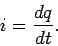(270)

Thus, the instantaneous current flowing around the circuit is numerically equal to the rate at which the charge accumulated on the positive plate of the capacitor increases with time. Equations (269) and (270) can be combined together to give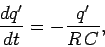(271)

where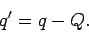(272)

At, just after the switch is closed, the charge on the positive plate of the capacitor is zero, so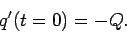(273)

Integration of Eq. (271), subject to the boundary condition (273), yields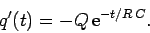(274)

It follows from Eq. (272) that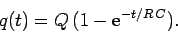(275)

The above expression specifies the chargeon the positive plate of the capacitor a time intervalafter the switch is closed (at time). The variation of the charge with time is sketched in Fig. 50. It can be seen that when the switch is closed the chargeon the positive plate of the capacitor does not suddenly jump up to its final value,. Instead, the charge increases smoothly from zero, and gradually asymptotes to its final value. The charge has risen to approximately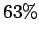of its final value a time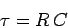(276)

after the switch is closed. By the time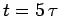, the charge has risen to more than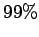of its final value. Thus,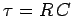is a good measure of how long after the switch is closed it takes for the capacitor to fully charge up. The quantity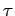is termed the time-constant, or thetime, of the circuit.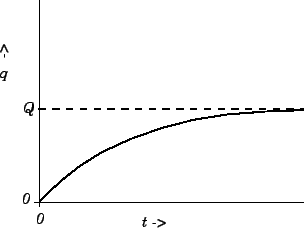According to Eqs. (270) and (271),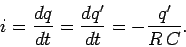(277)

It follows from Eq. (274) that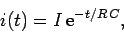(278)

where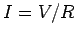. The above expression specifies the currentflowing around the circuit a time intervalafter the switch is closed (at time). It can be seen that, immediately after the switch is thrown, the currentwhich flows in the circuit is that which would flow if the capacitor were replaced by a conducting wire. However, this current is only transient, and rapidly decays away to a negligible value. After onetime, the current has decayed to 37% of its initial value. After fivetimes, the current has decayed to less than 1% of its initial value. It is interesting to note that for a short instant of time, just after the switch is closed, the current in the circuit acts as if there is no insulating gap between the capacitor plates. It essentially takes antime for the information about the break in the circuit to propagate around the circuit, and cause the current to stop flowing.

Suppose that we take a capacitor of capacitance, which is charged to a voltage, and discharge it by connecting a resistor of resistanceacross its terminals at. How long does it take for the capacitor to discharge? By analogy with the previous analysis, the chargeon the positive plate of the capacitor at timeis given by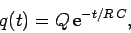(279)

whereis the initial charge on the positive plate. It can be seen that it takes a fewtimes for the capacitor to fully discharge. The currentwhich flows through the resistor is(280)

whereis the initial current. It can be seen that the capacitor initially acts like a battery of emf(since it drives the currentacross the resistor), but that, as it discharges, its effective emf decays to a negligible value on a fewtimes.Next: Transformers Up: Inductance Previous: The Circuit
Richard Fitzpatrick 2007-07-14